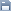Leetcode
2020.04.14 14:20

# 2. Add Two Numbers

조회 수 90 추천 수 0 댓글 0
?

#### 단축키

Prev이전 문서

Next다음 문서

크게 작게 위로 아래로 댓글로 가기 인쇄
?

#### 단축키

Prev이전 문서

Next다음 문서

크게 작게 위로 아래로 댓글로 가기 인쇄

You are given two non-empty linked lists representing two non-negative integers. The digits are stored in reverse order and each of their nodes contain a single digit. Add the two numbers and return it as a linked list.

You may assume the two numbers do not contain any leading zero, except the number 0 itself.

Example:

```Input: (2 -> 4 -> 3) + (5 -> 6 -> 4)
Output: 7 -> 0 -> 8
Explanation: 342 + 465 = 807.```

```/**
* Definition for singly-linked list.
* public class ListNode {
*     int val;
*     ListNode next;
*     ListNode(int x) { val = x; }
* }
*/
class Solution {
public ListNode addTwoNumbers(ListNode l1, ListNode l2) {
ListNode fake = new ListNode(0);
ListNode p = fake;

ListNode p1 = l1;
ListNode p2 = l2;

int carry = 0;

while(p1 != null || p2 != null){
int sum = carry;
if(p1!=null){
sum += p1.val;
p1 = p1.next;
}

if(p2!=null){
sum += p2.val;
p2 = p2.next;
}

if(sum>9){
carry=1;
sum = sum-10;
}else{
carry = 0;
}

ListNode l = new ListNode(sum);
p.next = l;
p = p.next;
}

//don't forget check the carry value at the end
if(carry > 0){
ListNode l = new ListNode(carry);
p.next = l;
}
return fake.next;
}
}```

?

 제목+내용제목내용댓글이름닉네임아이디태그
List of Articles
번호 분류 제목 글쓴이 날짜 조회 수
74 Leetcode 692. Top K Frequent Words 2020.04.15 87
» Leetcode 2. Add Two Numbers 2020.04.14 90
72 Leetcode 731. My Calendar II 2020.04.15 94
71 Leetcode 75. Sort Colors 2020.04.14 95
70 Leetcode 347. Top K Frequent Elements 2020.04.14 96
69 Leetcode 430. Flatten a Multilevel Doubly Linked List2020.04.06 98
68 Leetcode 23. Merge k Sorted Lists 2020.04.14 98
67 Leetcode 729. My Calendar I 2020.04.15 98
66 Leetcode 253. Meeting Rooms II 2020.04.15 98
65 Leetcode 20. Valid Parentheses 2020.04.25 98
64 Leetcode 62. Unique Paths2020.04.09 99
63 Leetcode 7. Reverse Integer 2020.04.28 100
62 Leetcode 946. Validate Stack Sequences 2020.04.08 101
61 Leetcode 973. K Closest Points to Origin 2020.04.15 101
60 Leetcode 852. Peak Index in a Mountain Array 2020.04.28 101
59 Leetcode 350. Intersection of Two Arrays II 2020.04.28 102
58 Leetcode 443. String Compression 2020.04.25 103
57 Leetcode 38. Count and Say 2020.04.25 103
56 Leetcode 53. Maximum Subarray 2020.04.28 106
55 Leetcode 3. Longest Substring Without Repeating Characters 2020.04.09 107
Board Pagination Prev 1 2 3 4 Next
/ 4